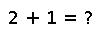# setdiff

setdiff(s1,s2)

Construct the set of elements in `s1` but not `s2`. Maintains order with arrays. Note that both arguments must be collections, and both will be iterated over. In particular, `setdiff(set,element)` where `element` is a potential member of `set`, will not work in general.

## Examples

``````julia> setdiff(Set(1,2,3,4),Set(2,3,5))
Set{Int64}({4,1})``````
``````julia> setdiff(Set(2,3),Set(2,3))
Set{Int64}({})``````
``````julia> all = [10:-1:1];
julia> odd = [1:2:10];

julia> even = setdiff(all, odd)     # note the ordering of elements in result
5-element Array{Int64,1}:
10
8
6
4
2``````

Checking you are not a robot: